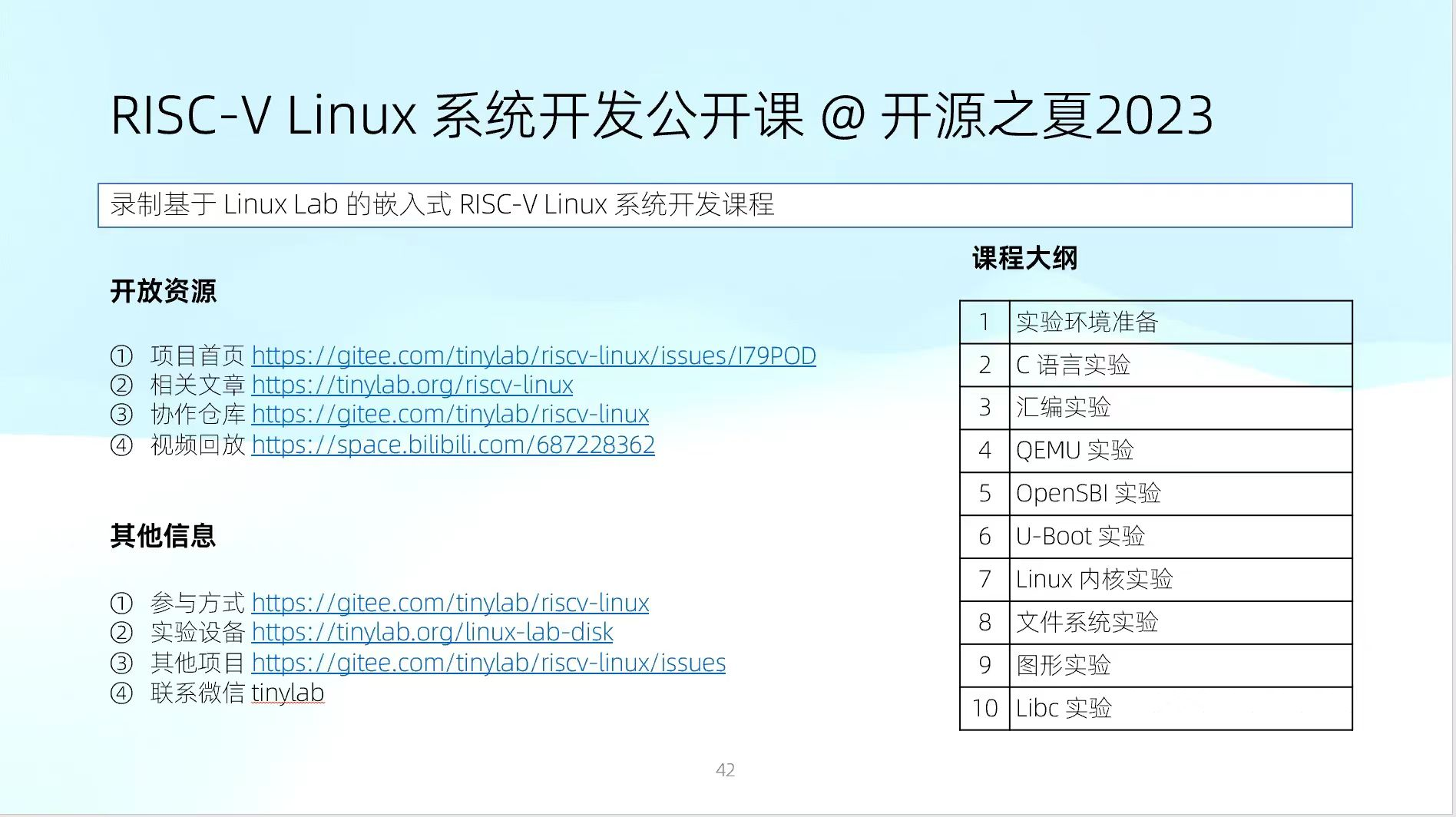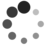# 通过操作 Section 为 Linux ELF 程序新增数据

By Falcon of TinyLab.org Nov 15, 2019

## 背景介绍

Section 是 Linux ELF 程序格式的一种核心数据表达方式，用来存放一个一个的代码块、数据块（包括控制信息块），这样一种模块化的设计为程序开发提供了很大的灵活性。

## 通过内联汇编新增一个 Section

``````asm(".pushsection .interp,\"a\"\n"
"        .string \"/lib/i386-linux-gnu/ld-linux.so.2\"\n"
".popsection");
``````

• `asm` 括号内就是汇编代码，这些代码几乎会被“原封不动”地放到汇编语言中间文件中（hello.s）。
• 这里采用 `.pushsection`, `.popsection`，而不是 `.section` 是为了避免之后的代码或者数据被错误地加到这里新增的 Section 中来。
• `.pushsection .interp, "a"`，这里的 “a” 表示 Alloc，会占用内存，这种才会被加到程序头表中，因为程序头表会用于创建进程映像。
• `.string` 这行用来指定动态链接器的完整路径。

• `.string` 可以替换为 `.incbin file`，然后把字符串内容放到名为 file 的文件中。文件末尾记得改为 `\0` 字节，可以用二进制编辑工具修改。
• `.string` 还可以替换为 `.ascii`，不过呢，末尾得主动加个 `\0` 字节，用法如下：
``````".ascii \"/lib/i386-linux-gnu/ld-linux.so.2\\x00\"\n"
``````

`.incbin` 方式在 Linux 内核中用处相当广泛，例如：

• `arch/arm/boot/bootp/kernel.S: .incbin "arch/arm/boot/zImage"`
• `kernel/configs.c: .incbin \"kernel/config_data.gz\"`

``````#include <stdio.h>
#include <unistd.h>

#if 1
asm(".pushsection .interp,\"a\"\n"
"        .ascii \"/lib/i386-linux-gnu/ld-linux.so.2\\x00\"\n"
".popsection");

/* .ascii above equals to .string \"/lib/i386-linux-gnu/ld-linux.so.2\"\n */
#else
asm(".pushsection .interp,\"a\"\n"
"        .incbin \"interp.section.txt\"\n"
".popsection");
#endif

int main(void)
{
printf("hello\n");

return 0;
}

void _start(void)
{
int ret;

ret = main();
_exit(ret);
}
``````

``````\$ gcc -m32 -shared -fpic -o libhello.so hello.c
\$ ./libhello.so
hello
``````

## 通过 gcc `__attribute__` 新增一个 Section

``````const char interp[] __attribute__((section(".interp"))) = "/lib/i386-linux-gnu/ld-linux.so.2";
``````

``````#include <stdio.h>
#include <unistd.h>

const char interp[] __attribute__((section(".interp"))) = "/lib/i386-linux-gnu/ld-linux.so.2";

int main(void)
{
printf("hello\n");

return 0;
}

void _start(void)
{
int ret;

ret = main();
_exit(ret);
}
``````

## 通过 objcopy 把某文件内容新增为一个 Section

objcopy 这个工具很强大，其中就包括新增 Section。

``````\$ echo -e -n "/lib/i386-linux-gnu/ld-linux.so.2\x00" > interp.section.txt
``````

``````#include <stdio.h>
#include <unistd.h>

int main(void)
{
printf("hello\n");

return 0;
}

void _start(void)
{
int ret;

ret = main();
_exit(ret);
}
``````

``````\$ objcopy --add-section .interp=interp.section.txt --set-section-flags .interp=alloc,readonly libhello.so
objcopy:stTyWnxc: can't add section '.interp': File in wrong format
``````

``````\$ gcc -m32 -shared -fpic -c -o hello.o hello.c
\$ gcc -m32 -shared -fpic -o libhello.so hello.o
``````

## 通过 objcopy 更新某个 Section

``````\$ dpkg -S /lib/i386-linux-gnu/ld-linux.so.2
libc6:i386: /lib/i386-linux-gnu/ld-linux.so.2
``````

``````\$ dpkg -S /lib32/ld-linux.so.2
libc6-i386: /lib32/ld-linux.so.2
``````

``````\$ objcopy --dump-section .interp=interp.txt libhello.so
\$ sed -i -e "s%/lib/i386-linux-gnu/ld-linux.so.2%/lib32/ld-linux.so.2%g" interp.txt
\$ objcopy --update-section .interp=interp.txt libhello.so
\$ ./libhello.so
hello
``````